Question-and-Answer Resource for the Building Energy Modeling Community
Get started with the Help page

# Where are the default chiller curve coefficient coming from in OS?

As the name implies, I'm trying to understand what's the rationale behind the default curve that come with the chiller

Let's take for example a Chiller:Electric:EIR and look specifically at the EIRFPLR curve, that is the Electric Input Ratio as a function of the Part Load Ratio.

This curve is quadratic: $$EIR = a + b\times PLR + c \times PLR^2$$

OpenStudio has the following default coefficients: - $a = 0.06369119$, - $b = 0.58488832$ - $c = 0.35280274$.

It seems that it leads to a pretty efficient chiller, unless I'm missing something. I've compared it t:

• the Comnet modeling guidelines here (Table 6.8.2-9: "Default Efficiency EIR-FPLR Coefficients – Water-Cooled Chillers" > Centrifugal)

• DOE-2 Centrifugal/5.50COP from the E+ dataset (EnergyPlusV8-3-0\DataSets\Chillers.idf)

a b c
Comnet 0.171493 0.588202 0.237373
DOE-2 Centrifugal COP 5.50 0.222903 0.313387 0.463710
OpenStudio 0.063691 0.584888 0.352803

I end up with the following curves where I'm displaying COP (SI units) instead of EIR since it's more intuitive in my opinion.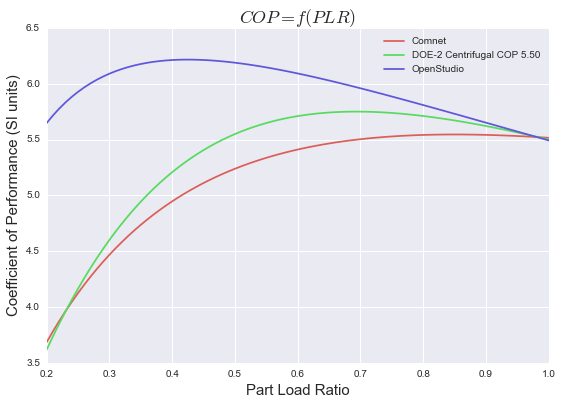Anyone can explain why it seems so much more efficient than the rest?

edit retag close merge delete

How are you arriving at your COP number 'in SI units'? COP is a dimensionless value, it's a measure of energy produced divided by energy consumed.

COP in W/W. I just specified SI Units because I've seen confusion about it before, where people would use the word COP when it was in fact an EER ($BTU_{out}/W_{in}$)

Bonus point: which curve (from the E+ dataset for example) one would use for a centrifugal water cooled chiller? Let's say per ASHRAE 90.1-2010. I think this might warrant a question of its own, since they only give you a rated COP and an IPLV to match.

Sort by » oldest newest most voted

The OpenStudio default chiller coefficients correspond with those published in the Title 24 Nonresidential ACM for air-cooled scroll chillers (page 2-98 here), as well as the "Minimum Efficiency Standards" appendix for California Utility incentive programs, which states the coefficients come from a California Energy Commission study.

From footnote 8 in this paper from Taylor Engineering, it appears these coefficients were first published in the first public review of ASHRAE Standard 90.1-1989 (March, 1996). However I haven't found this document to ascertain specifically how the coefficients were derived.

more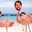@Julien Marrec, I don't think the COPs have been calculated correctly from the given coefficients because when I take the same coefficients, calculate the COPs and plot them I get the image below.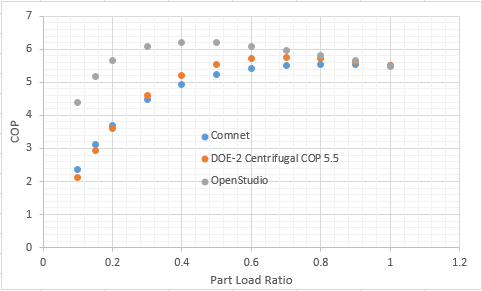I created a test model with a chiller and used the OpenStudio curves to verify the results and viewed them in ResultsViewer. The first image is the part load ratio hourly and we'll use a reference point with a PLR of 0.2 to then look at the EIRFT and COP. At a PLR of 0.2 you I would expect a COP of 5.64 as shown in the first image above, but also at a PLR of 0.2 the EIRFT = 1.08569 and thus the actual COP for the given conditions would be 5.64/1.08 = 5.20 as can be seen in the third image below.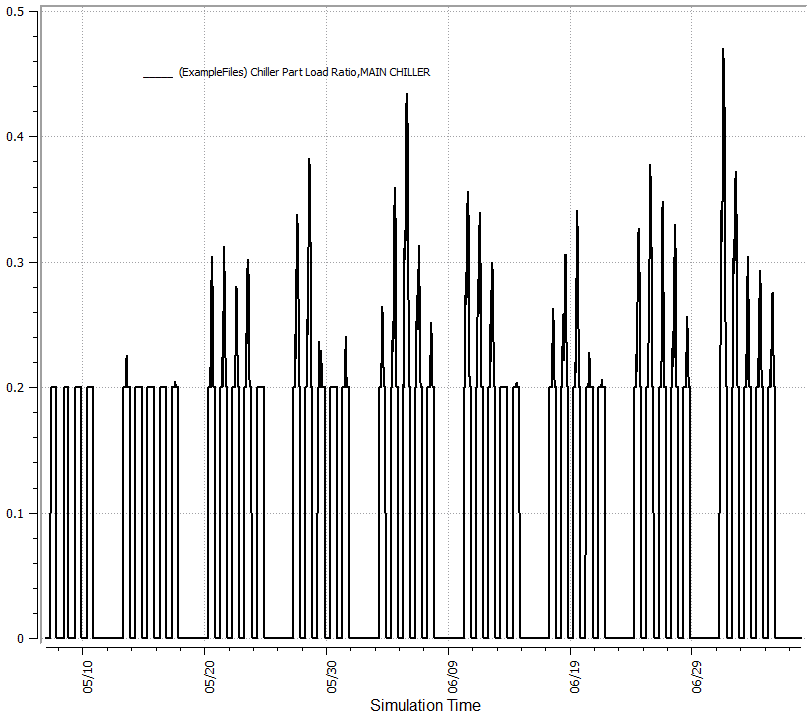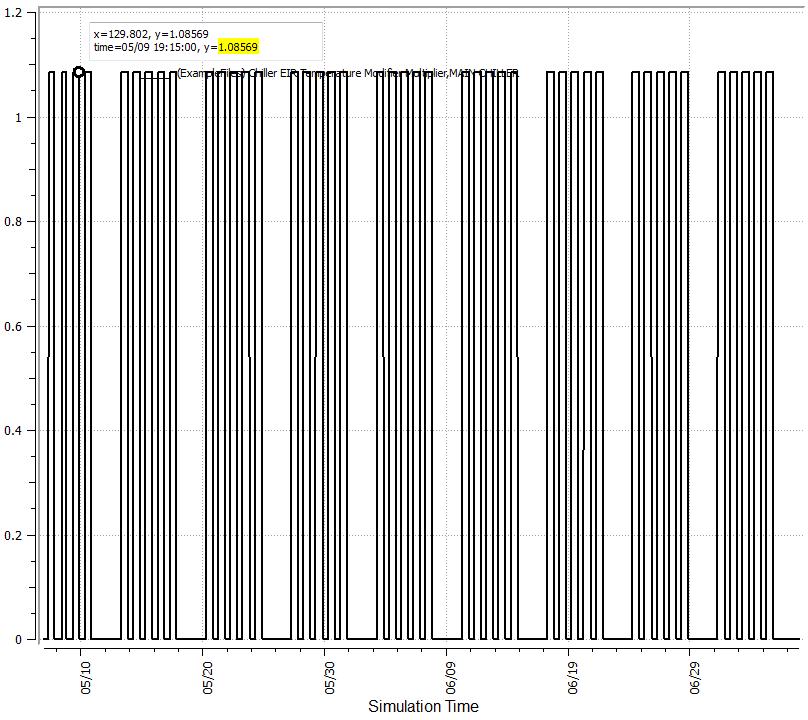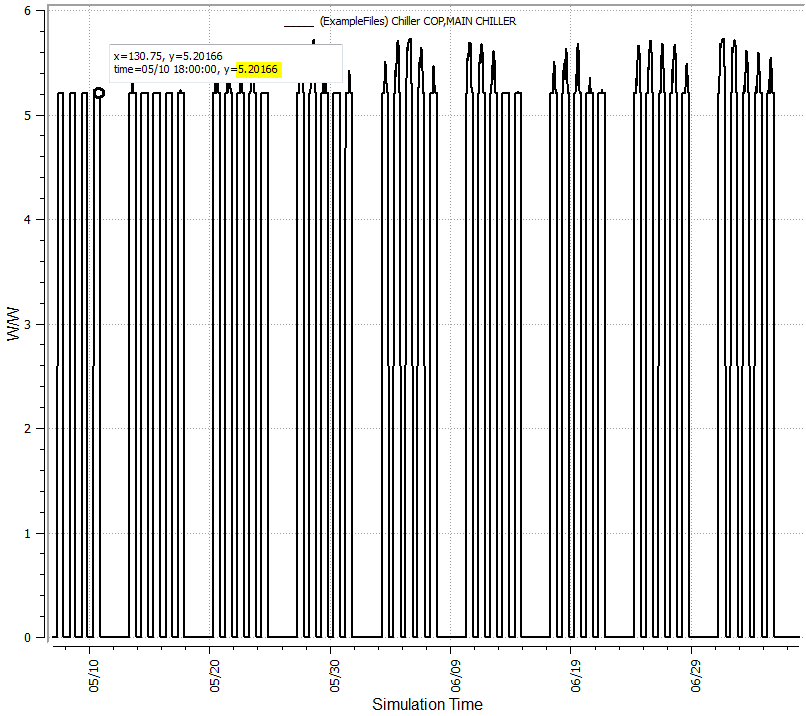It looks like what you have done is calculated the EIRFPLR, but have not divided through by the PLR to get the correct EIR adjustment factor. To get the COP you first need to calculate, assuming a quadratic, the following:

$$EIRFPLR = a + b \times PLR + c \times PLR^2$$

The EIRFPLR value is then divided through by the PLR to get an EIR adjustment factor, which then gets multiplied by the full load EIR to get the actual EIR for a specific part load. As you know, the COP is just the inverse of this.

$$EIR_{actual} = \frac{EIRFPLR \times EIR_{ref}}{PLR}$$

The variance in the curves is probably due to the type of chiller the coefficients are representing. The Comnet and DOE-2 curves looks more similar to a reciprocating chiller despite DOE-2 saying centrifugal where as the OpenStudio default looks more like a scroll chiller.

UPDATE

After reading what I wrote I realized I could make it a bit clearer. For the following segment I will use $hr$ to denote timestep values of variables and $n$ for the rated nominal capacity.

• $CAP$ = capacity
• $PLR$ = part load ratio
• $P$ = power

The capacity for a given timestep can be found as $CAP_{hr} = CAP \times CAPFT$ and power, $P_{hr}$, is expressed as:

$$P_{hr} = CAP_{hr} \times EIR_{n} \times EIRFT \times EIRFPLR$$

Assuming $EIRFT$ is at rated conditions and thus equals 1 we get:

$$P_{hr} = CAP_{hr} \times EIR_{n} \times EIRFPLR$$

$$\frac{P_{hr}}{CAP_{hr}} = EIR_{n} \times EIRFPLR$$

However, we don't know $CAP_{hr}$, but if we substitute in $CAP_{hr} = \frac{LOAD_{hr}}{PLR}$ then we get:

$$P_{hr} \times \frac{PLR}{LOAD_{hr}} = EIR_{n} \times EIRFPLR$$

$$\frac{P_{hr}}{LOAD_{hr}} = EIR_{hr}$$

$$EIR_{hr} \times PLR = EIR_{n} \times EIRFPLR$$

Seeing as we want $EIR_{hr}$ we can rearrange and have all the variables known on one side and represent it as

$$EIR_{hr} = \frac{EIR_{n} \times EIRFPLR}{PLR}$$

more

Jesus... I did forget to divide by the PLR. It's such an obvious mistake. Thanks a ton... I'll edit my question to have a proper graph (and the question still remains). I'll also add a little bit of MathJax to format your above equations

I marked Eric's answer has accepted as it answers the question, which is "where is it coming from?", but if I could mark two I'll definitely give you the karma too. For now you'll have to do with my upvote and gratitude.

Yup, that's fair. I was just trying to clear up some of what was missing from the original post and I didn't actually have an answer to your main question.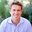The problem is that OpenStudio doesn't change the performance curves when you go from air-cooled to water-cooled. The user must change these manually.

more

Ultimately I would like to see the day where we have a wide range of "real" products from TPEX. I think that will minimize this sort of issue. Until then shall we add a distinct air cooled and water cooled instance to OS's built in hvac library? I'd be happy to put it in there if someone from this thread can provide the content.

Would it be possible to add one additional user-defined field? The field would allow the user to define the chiller compressor type (Scroll, Recip, Screw, Centrifugal). Based on this selection and the Condenser Type selection the proper curve could be determined based on Tables 6.8.2-3 and 6.8.2-4 from the Comnet modeling guidelines.

This means there would need to be 7 different curves added to the OpenStudio built-in HVAC Library. 3 different air-cooled curves and 4 different water-cooled curves. It may be asking a lot but it will keep people out of trouble.

1

I like where this is going. I think we need something along these lines to do standards properly anyway. What you describe gets tricky. The hvac library currently doesn't have any room for logic, so the dropping in of the proper curve based on the proposed new field could not happen automagically. We could simply put in all combinations of chiller in the hvac library, then user would have to drag and drop the fully fleshed out chiller with all properties in sync. I don't think this is what you are getting at though.

I have been kicking around a new hvac library architecture that is based on OpenStudio Measure, and this would allow for "logic on drop." Gotta do something here, because the current hvac library is not maintainable or scalable. Interested in keeping this conversation going. I'm a bit tied up in 1.9 features at the moment, but maybe a phone call at some point in the future would be more expedient.

Ah I did not realize that the OS HVAC library was so static. Give me a call when you have some time.